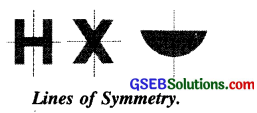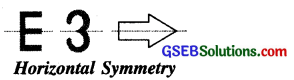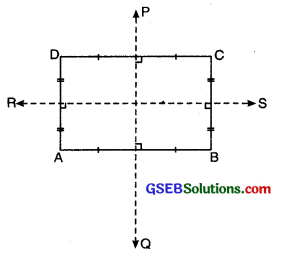# GSEB Class 6 Maths Notes Chapter 13 Symmetry

This GSEB Class 6 Maths Notes Chapter 13 Symmetry covers all the important topics and concepts as mentioned in the chapter.

## Symmetry Class 6 GSEB Notes

Introduction:
Symmetry is quite a common term used in day to day life. When we see certain figures with evenly balanced proportions, we say, “They are symmetrical.”

Symmetry is an important geometrical concept exhibited in nature. It is widely used in engineering, architecture, textile designing, pottery and many other fields. We can observe symmetry in nature such as flowers, leaves and insects. We find symmetry on bed sheets, shawls and also in monuments like Taj Mahal and Eiffel Tower.

Symmetry:
When a picture is folded from the middle and the two halves match exactly, then we say that the picture is symmetrical. This phenomenon is called Symmetry.Lines of Symmetry:
A figure is said to hav&a line symmetry if on folding the figure about this line, the two parts of the figure coincide.
If we observe the symmethcal figure, there are some lines which divide the figure into two exactly identical halves. These lines are called lines of symmetry or axis of Symmetry.Let us observe the pictures shown in Fig. The line of symmetry in these pictures is horizontal. This type of symmetry is called horizontal symmetry.Let us observe the pictures shown in Fig. The line of symmetry in these pictures is vertical. This type of symmetry is called vertical symmetry.Two Lines of Symmetry. Take a rectangle ABCD when it is folded about PQ (the perpendicular bisectors of AB and CD), the two parts exactly coincide.Similarly, when folded about RS, the two parts coincide exactly. But if we try to fold it about any other line, we find that the two parts do not coincide.

So we can say that a rectangle has two lines of symmetry.
Similarly, we can discuss more figures having two lines of symmetry.

Multiple Lines of Symmetry
We come across some figures which have more than two lines of symmetry.
For Example. A square has four lines of symmetry.
An equilateral triangle has three lines of symmetry.
A circle has infinite lines of symmetry.Reflection Symmetry
The object and its image are symmetrical with reference to the mirror line. The image of the object is the reflection of the object in the mirror line. When an object is reflected, there is no change in lengths and angles i.e. the lengths and angles of the object and the corresponding lengths and angles of the image are the same.